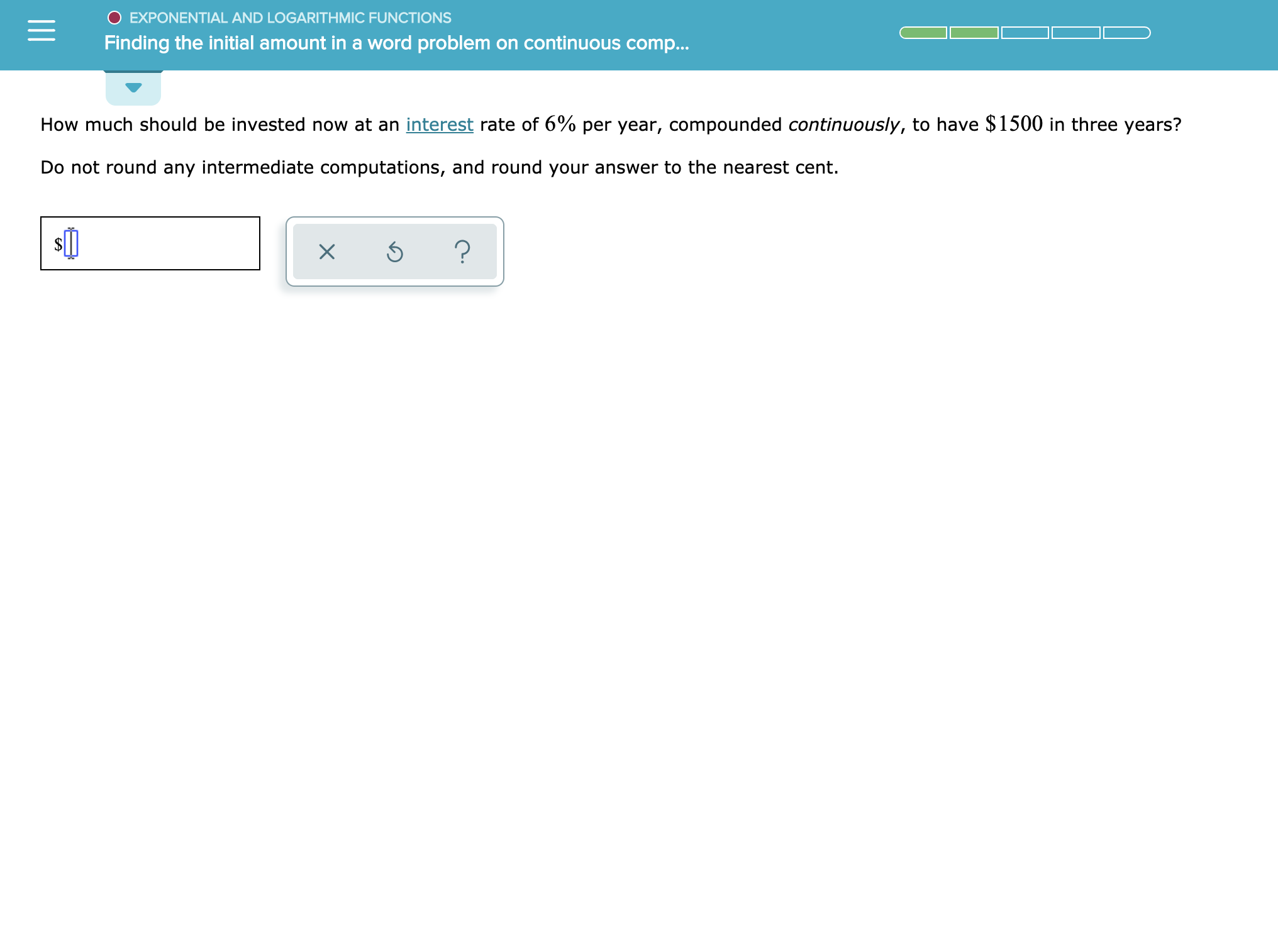# O EXPONENTIAL AND LOGARITHMIC FUNCTIONSFinding the initial amount in a word problem on continuouse comp...How much should be invested now at an interest rate of 6% per year, compounded continuously, to have \$1500 in three years?Do not round any intermediate computations, and round your answer to the nearest cent.?

Question
24 views

See attachmenthelp_outlineImage TranscriptioncloseO EXPONENTIAL AND LOGARITHMIC FUNCTIONS Finding the initial amount in a word problem on continuouse comp... How much should be invested now at an interest rate of 6% per year, compounded continuously, to have \$1500 in three years? Do not round any intermediate computations, and round your answer to the nearest cent. ? fullscreen
check_circle

Step 1

To determine the amount that should be invested to get a return of \$ 1500 in three provided that the rate of interest is 6% per year compounded continuously.

Step 2

The accumulated amount A after t years for principal amount P invested at a rate of interest r compounded continuously is given by the formula:

Step 3

Consider that the rate of interest is 6%, accumulated amount...

### Want to see the full answer?

See Solution

#### Want to see this answer and more?

Solutions are written by subject experts who are available 24/7. Questions are typically answered within 1 hour.*

See Solution
*Response times may vary by subject and question.
Tagged in

### Other